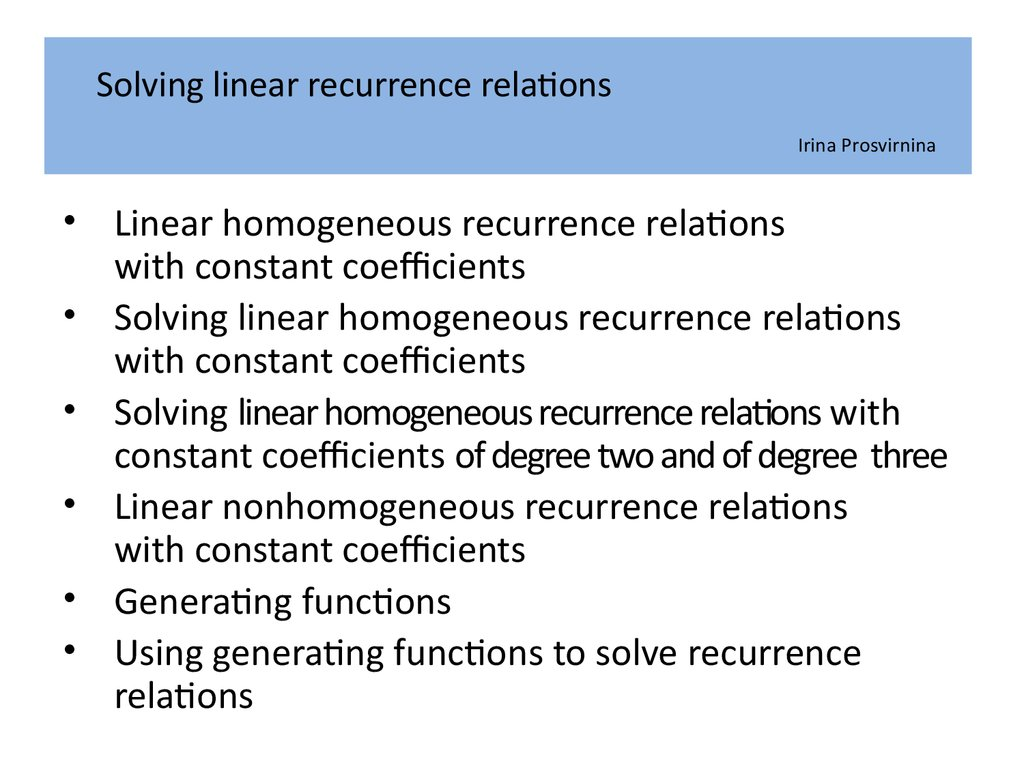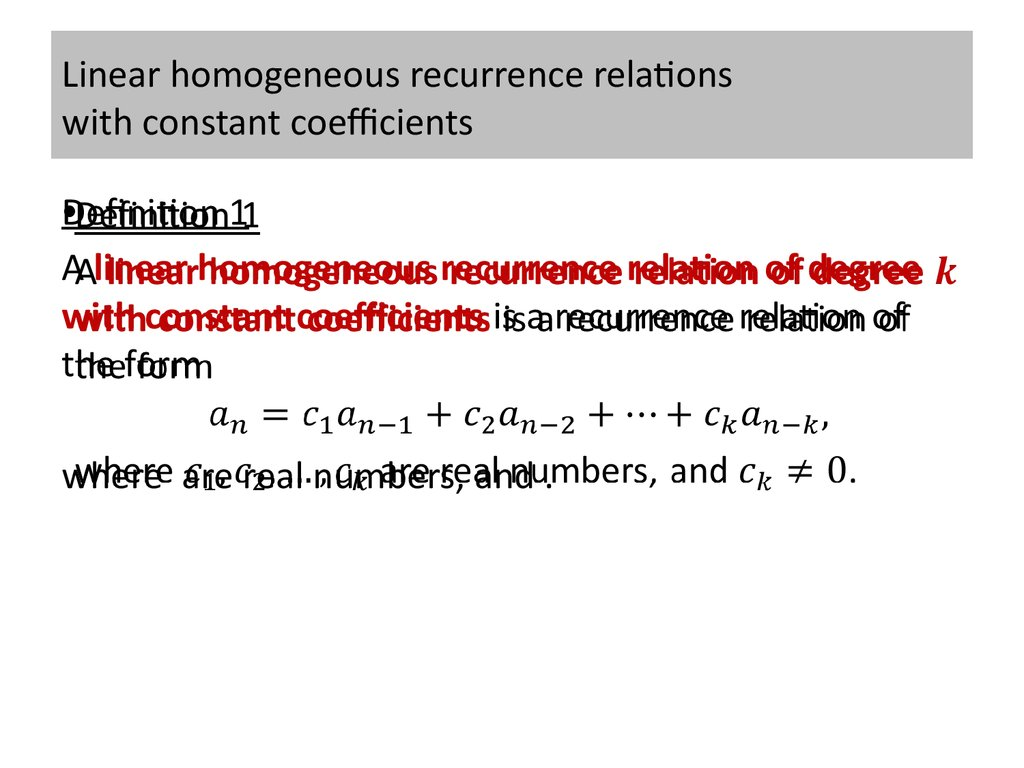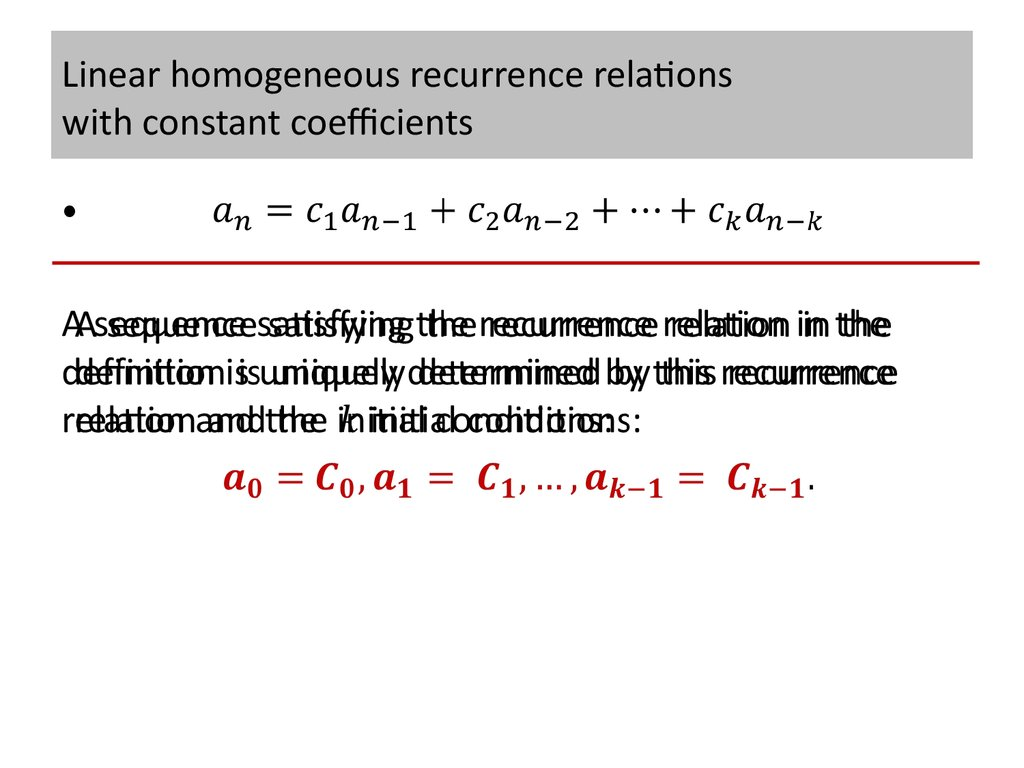# Solving linear recurrence relations

## 1. Solving linear recurrence relations Irina Prosvirnina

• Linear homogeneous recurrence relations
with constant coefficients
• Solving linear homogeneous recurrence relations
with constant coefficients
• Solving linear homogeneous recurrence relations with
constant coefficients of degree two and of degree three
• Linear nonhomogeneous recurrence relations
with constant coefficients
• Generating functions
• Using generating functions to solve recurrence
relations

## 2. Linear homogeneous recurrence relations with constant coefficients

•Definition 1
A linear homogeneous recurrence relation of degree
with constant coefficients is a recurrence relation of
the form
where are real numbers, and .

## 3. Linear homogeneous recurrence relations with constant coefficients

A sequence satisfying the recurrence relation in the
definition is uniquely determined by this recurrence
relation and the initial conditions:

## 4. Linear homogeneous recurrence relations with constant coefficients

•Example 1
The recurrence relation
is a linear homogeneous recurrence relation of degree
one.

## 5. Linear homogeneous recurrence relations with constant coefficients

•Example 2
The recurrence relation
is a linear homogeneous recurrence relation of degree
two.
The sequence of Fibonacci numbers satisfies this
recurrence relation and also satisfies the initial
conditions .

## 6. Linear homogeneous recurrence relations with constant coefficients

•Example 3
The recurrence relation
is a linear homogeneous recurrence relation of degree
five.

## 7. Linear homogeneous recurrence relations with constant coefficients

•Example 4
The recurrence relation
is not linear.

## 8. Linear homogeneous recurrence relations with constant coefficients

•Example 5
The recurrence relation
is not homogeneous.

## 9. Linear homogeneous recurrence relations with constant coefficients

•Example 6
The recurrence relation
does not have constant coefficients.

## 10. Solving linear homogeneous recurrence relations with constant coefficients

•The basic approach for solving linear homogeneous
recurrence relations
is to look for solutions of the form
where is a constant.

## 11. Solving linear homogeneous recurrence relations with constant coefficients

•Note that
is a solution of the recurrence relation
if and only if

## 12. Solving linear homogeneous recurrence relations with constant coefficients

When both sides of this equation are divided by , we
obtain the equation
When the right-hand side is subtracted from the left we
obtain the equation

## 13. Solving linear homogeneous recurrence relations with constant coefficients

Consequently,
the sequence with is a solution of linear
homogeneous recurrence relations
with constant coefficients
is a solution if and only if
is a solution of this last equation
We call this the characteristic equation of the recurrence
relation .
The solutions of this equation are called the
characteristic roots of the recurrence relation .

## 14. Solving linear homogeneous recurrence relations with constant coefficients

As we will see, these characteristic roots can be used to
give an explicit formula for all the solutions of the
recurrence relation.

## 15. Solving linear homogeneous recurrence relations with constant coefficients of degree two

Theorem
1
Let and be real numbers. Suppose that
has two distinct roots and .
Then the sequence is a solution of the recurrence
relation
if and only if for , where and are constants.

## 16. Proof of theorem 1

?• If and are roots of , and are constants then the
sequence with is a solution of the recurrence relation
.

## 17. Proof of theorem 1

?• If the sequence is a solution of , then for , for some
constants and , where and are distinct roots of .
Let is a solution of the recurrence relation
and the initial conditions hold.

## 18. Proof of theorem 1

?• If the sequence is a solution of , then for , for some
constants and , where and are distinct roots of .
It will be shown that there are constants and such that
the sequence with satisfies these same initial
conditions

## 19. Proof of theorem 1

?• If the sequence is a solution of , then for , for some
constants and , where and are distinct roots of .
This requires that
We can solve these two equations for and :

## 20. Proof of theorem 1

?• If the sequence is a solution of , then for , for some
constants and , where and are distinct roots of .
Hence, with these values for
the sequence with ,
satisfies the two initial conditions

## 21. Proof of theorem 1

?• If the sequence is a solution of , then for , for some
constants and , where and are distinct roots of .
We know that and are both solutions of the recurrence
relation and both satisfy the initial conditions when
and .
Because there is a unique solution of a linear
homogeneous recurrence relation of degree two with
two initial conditions, it follows that the two solutions
are the same, that is, for .

## 22. Solving linear homogeneous recurrence relations with constant coefficients of degree two

•Example 7
What is the solution of the recurrence relation
with and ?
Solution
The characteristic equation of the recurrence relation is
Its roots are
and .
By theorem 1, .

## 23. Solving linear homogeneous recurrence relations with constant coefficients of degree two

•Example 7
What is the solution of the recurrence relation
with and ?
Solution

## 24. Solving linear homogeneous recurrence relations with constant coefficients of degree two

•Example 8 (Fibonacci numbers)
What is the solution of the recurrence relation
with and ?
Solution
The characteristic equation of the recurrence relation is
Its roots are
and .
By theorem 1,

## 25. Solving linear homogeneous recurrence relations with constant coefficients of degree two

•Example 8 (Fibonacci numbers)
What is the solution of the recurrence relation
with and ?
Solution

## 26. Solving linear homogeneous recurrence relations with constant coefficients of degree two

Theorem
2
Let and be real numbers with . Suppose that
has only one root .
A sequence is a solution of the recurrence relation
if and only if for , where and are constants.

## 27. Solving linear homogeneous recurrence relations with constant coefficients of degree two

•Example 9
What is the solution of the recurrence relation
with and ?
Solution
The characteristic equation of the recurrence relation is
Its root is
.
By theorem 2,

## 28. Solving linear homogeneous recurrence relations with constant coefficients of degree two

•Example 9
What is the solution of the recurrence relation
with and ?
Solution

## 29. Solving linear homogeneous recurrence relations with constant coefficients of degree three

Theorem
3
Let be real numbers.
Suppose that the characteristic equation
has distinct roots .
A sequence is a solution of the recurrence relation
if and only if
for , where are constants.

## 30. Solving linear homogeneous recurrence relations with constant coefficients of degree three

•Example 10
What is the solution of the recurrence relation
with
Solution
The characteristic equation of the recurrence relation is
Its roots are
.
By theorem 3,

## 31. Solving linear homogeneous recurrence relations with constant coefficients of degree three

Example
10
What is the solution of the recurrence relation
with
Solution
.

## 32. Linear nonhomogeneous recurrence relations with constant coefficients

Definition
2
A linear nonhomogeneous recurrence relation with
constant coefficients is a recurrence relation of the
form
where are real numbers, is a function not identically
zero depending only on .
The recurrence relation
is called the associated homogeneous recurrence
relation.
It plays an important role in the solution of the
nonhomogeneous recurrence relation.

## 33. Linear nonhomogeneous recurrence relations with constant coefficients

•Example 11
The recurrence relation
is a linear nonhomogeneous recurrence relation with
constant coefficients.
The associated linear homogeneous recurrence relation
is
.

## 34. Linear nonhomogeneous recurrence relations with constant coefficients

•Example 12
The recurrence relation
is a linear nonhomogeneous recurrence relation with
constant coefficients.
The associated linear homogeneous recurrence relation
is
.

## 35. Linear nonhomogeneous recurrence relations with constant coefficients

•Example 13
The recurrence relation
is a linear nonhomogeneous recurrence relation with
constant coefficients.
The associated linear homogeneous recurrence relation
is
.

## 36. Linear nonhomogeneous recurrence relations with constant coefficients

•Example 14
The recurrence relation
is a linear nonhomogeneous recurrence relation with
constant coefficients.
The associated linear homogeneous recurrence relation
is
.

## 37. Linear nonhomogeneous recurrence relations with constant coefficients

Theorem
4
If is a particular solution of the nonhomogeneous
linear recurrence relation with
constant coefficients
,
then every solution is of the form ,
where is a solution of the associated
homogeneous recurrence relation

## 38. Proof of theorem 4

Because
is a particular solution of the
nonhomogeneous recurrence relation
,
we know that

## 39. Proof of theorem 4

Now
• suppose that is a second solution of the
nonhomogeneous recurrence relation
,
so that
.

## 40. Proof of theorem 4

So,
,
.
Subtracting the first of these two equations from the
second shows that
It follows that is a solution of the associated homogeneous
linear recurrence relation,say,
Consequently,

## 41. Linear nonhomogeneous recurrence relations with constant coefficients

•Example 15
Find all solutions of the recurrence relation
Solution
This is a linear nonhomogeneous recurrence relation.
The solutions of its associated homogeneous
recurrence relation
are

## 42. Linear nonhomogeneous recurrence relations with constant coefficients

•Example 15
Find all solutions of the recurrence relation
Solution
We now find a particular solution.
Suppose that where and are constants, such a
solution.

## 43. Linear nonhomogeneous recurrence relations with constant coefficients

•Example 15
Find all solutions of the recurrence relation
Solution

## 44. Linear nonhomogeneous recurrence relations with constant coefficients

•Example 16
Find all solutions of the recurrence relation
Solution
This is a linear nonhomogeneous recurrence relation.
The solutions of its associated homogeneous
recurrence relation
are .

## 45. Linear nonhomogeneous recurrence relations with constant coefficients

•Example 16
Find all solutions of the recurrence relation
Solution
We now find a particular solution.
Suppose that is a

## 46. Linear nonhomogeneous recurrence relations with constant coefficients

•Example 16
Find all solutions of the recurrence relation
Solution

## 47. Generating functions

•Definition 3
The generating function for the sequence
of real numbers is the infinite series

## 48. Generating functions

•Example 17
The generating function for the sequence
is

## 49. Generating functions

•Example 18
The generating function for the sequence
is

## 50. Generating functions

•Example 19
The generating function for the sequence
is

## 51. Generating functions

We
• can define generating functions for finite sequences
of real numbers by extending a finite sequence
into an infinite sequence by setting
and so on.
The generating function of this infinite sequence is a
polynomial of degree because no terms of the form
with occur, that is,

## 52. Generating functions

•Example 20
The generating function of
is
We have
when .
Consequently, is the generating function of the sequence

## 53. Generating functions

•Example 21
Let be a positive integer.
The generating function for the sequence
with
is
The binomial theorem shows that

## 54. Generating functions

•Example 22
The function
is the generating function of the sequence
because
for

## 55. Generating functions

•Example 23
The function
is the generating function of the sequence
because
for

## 56. Using generating functions to solve recurrence relations

•Example 24
Solve the recurrence relation
for
and initial condition

## 57. a_k=3a_(k-1), a_0=2

•Solution of example 24
Let be the generating function for the sequence , that
is,
First note that

## 58. a_k=3a_(k-1), a_0=2

•Solution of example 24

## 59. a_k=3a_(k-1), a_0=2

•Solution of example 24

## 60. a_k=3a_(k-1), a_0=2

•Solution of example 24

## 61. Using generating functions to solve recurrence relations

Example
25
Solve the recurrence relation
for
and initial condition
Suppose that a valid codeword is an -digit number in
decimal notation containing an even number of 0s.
Let denote the number of valid codewords of length .
Proof that satisfies the recurrence relation
and the initial condition
Use generating functions to find an explicit formula for .

## 62. a_n=8a_(n-1)+〖10〗^(n-1), a_1=9

•Solution of example 25
To make our work with generating functions simpler,
we extend this sequence by setting and use the
recurrence relation, we have
which is consistent with our original initial condition.
(It also makes sense because there is one code word of
length – the empty string.)

## 63. a_n=8a_(n-1)+〖10〗^(n-1), a_0=1

•Solution of example 25
Let
be the generating function of the sequence

## 64. a_n=8a_(n-1)+〖10〗^(n-1), a_0=1

•Solution of example 25
We sum both sides of the last equation starting with ,
to find that

## 65. a_n=8a_(n-1)+〖10〗^(n-1), a_0=1

•Solution of example 25

## 66. a_n=8a_(n-1)+〖10〗^(n-1), a_0=1

•Solution of example 25

## 67. a_n=8a_(n-1)+〖10〗^(n-1), a_0=1

•Solution of example 25
Expanding the right-hand side of this equation into
partial fractions gives

## 68. a_n=8a_(n-1)+〖10〗^(n-1), a_0=1

•Solution of example 25

## 69. a_n=8a_(n-1)+〖10〗^(n-1), a_0=1

•Solution of example 25